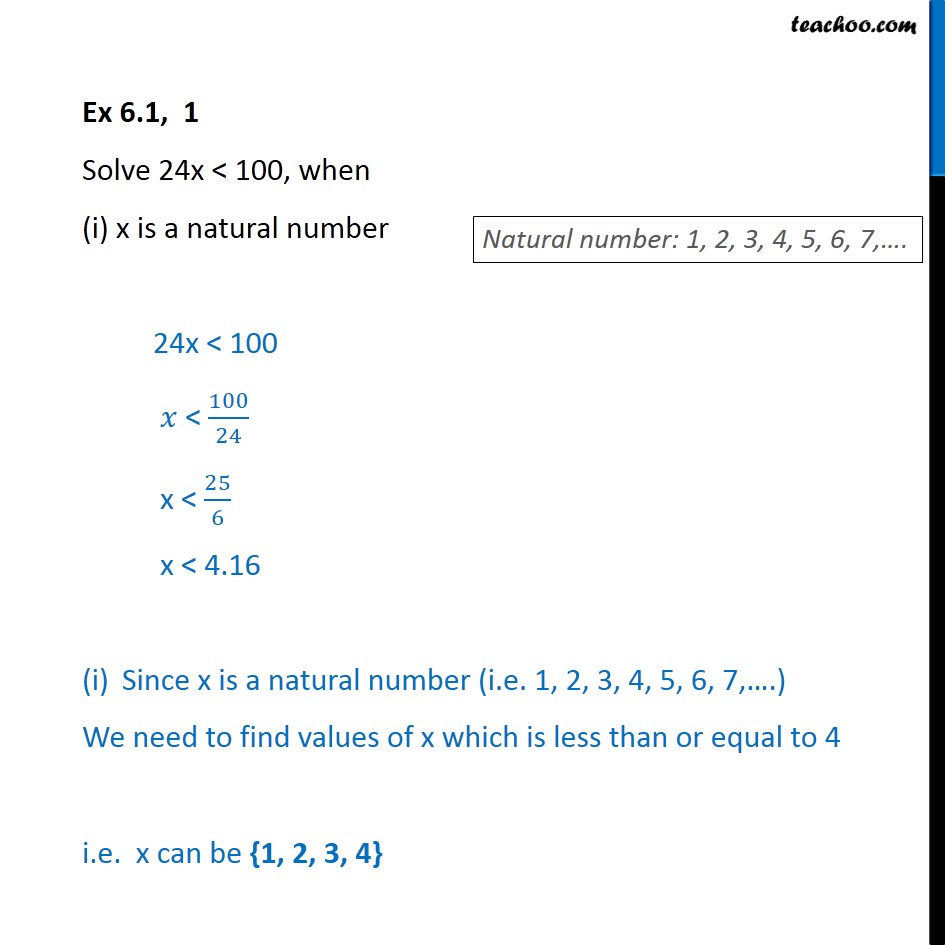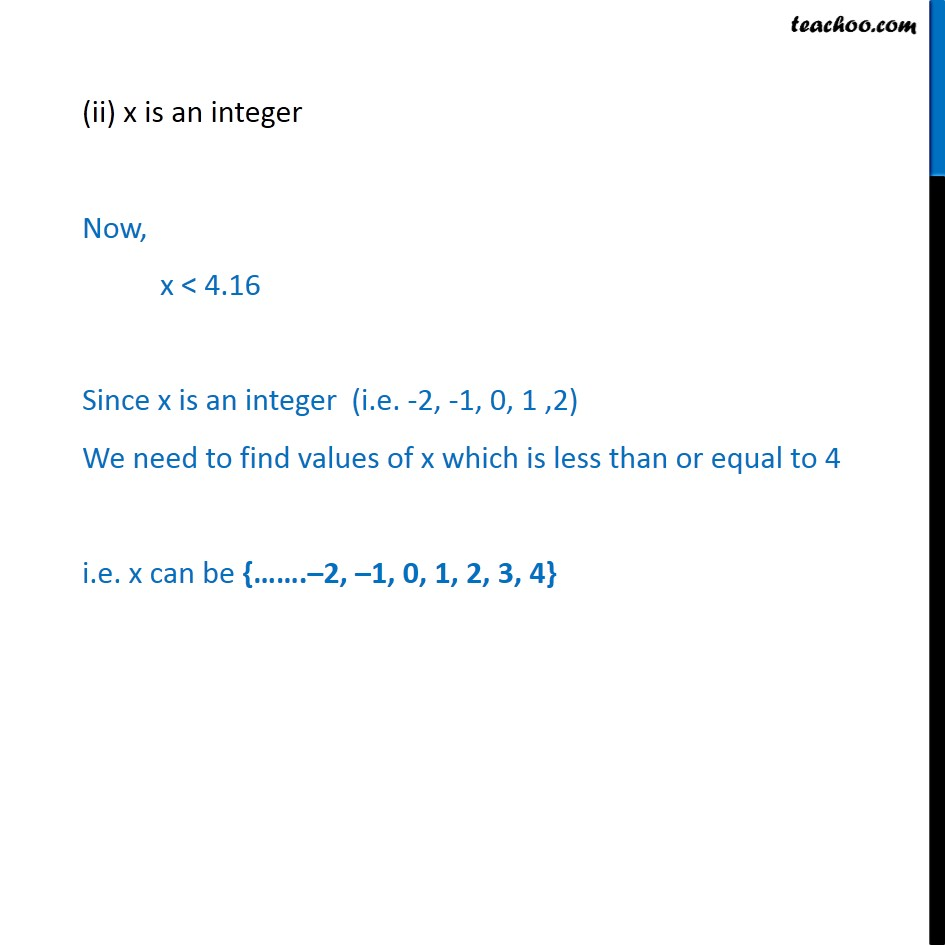1. Chapter 6 Class 11 Linear Inequalities (Term 2)
2. Serial order wise
3. Ex 6.1

Transcript

Ex 6.1, 1 Solve 24x < 100, when (i) x is a natural number 24x < 100 𝑥 < ﷐100﷮24﷯ x < ﷐25﷮6﷯ x < 4.16 Since x is a natural number (i.e. 1, 2, 3, 4, 5, 6, 7,….) We need to find values of x which is less than or equal to 4 i.e. x can be {1, 2, 3, 4} (ii) x is an integer Now, x < 4.16 Since x is an integer (i.e. -2, -1, 0, 1 ,2) We need to find values of x which is less than or equal to 4 i.e. x can be {…….–2, –1, 0, 1, 2, 3, 4}